# Seats for a dining table

We would like to illustrate the concept of comfort at the table that allows us to define the maximum number of seats depending on the size of the table. This can help in the choice of purchasing a table both for the house or public environments such as bars and restaurants, so the capacity of seats for each table can be optimized, preserving the comfort.

Below we illustrate the criteria by which we calculate the maximum number of seats for a table:

It should be remembered that by varying these criteria it is possible that the maximum number of seats may decrease, especially in the case of a table with a 4-legged base with a considerable thickness. Even the choice of a chair with armrests or a large armchair can affect the space available for seats.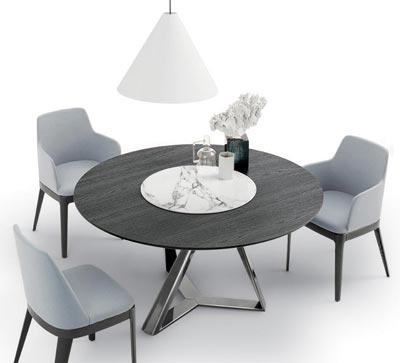## Rectangular tables

An extendable or fixed rectangular table has a short side of a minimum length of 70 cm and a long side that can reach even over 300 cm. Tables with a short side greater than or equal to 90 cm are suitable for placing more glasses, bottles and any serving dishes.

To know the number of seats for a rectangular dining table the calculation is very simple. On the short side we consider that the minimum space for a diner is 70 cm, while for the long side the space needed for a diner is 60 cm.

Let's take for example a rectangular table 130x90 cm.

• 130 / 60 = 2.16 which we round to 2
• 90 / 70 = 1.28 which we round to 1
• Number of seats = 2 + 2 + 1 + 1 = 6

In rectangular tables with a short side of 70 cm we prefer to use another criterion to preserve the comfort of the guests. In this case we consider the minimum footprint on all sides of 70 cm.

Let's take for example a rectangular table 130x70 cm.

• 130 / 70 = 1.80 which we round to 1
• 70 / 70 = 1
• Number of seats = 1 + 1 + 1 + 1 = 4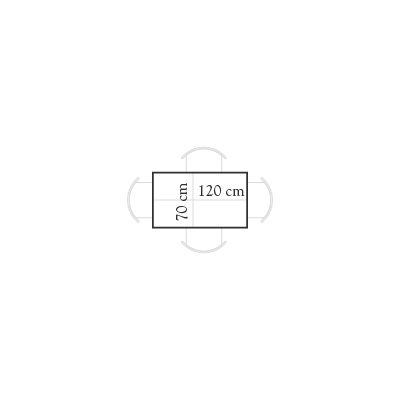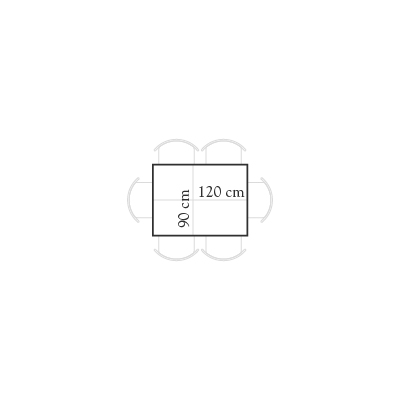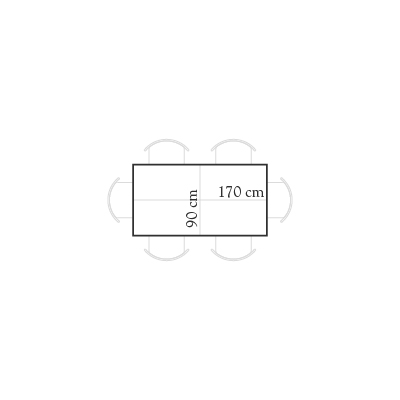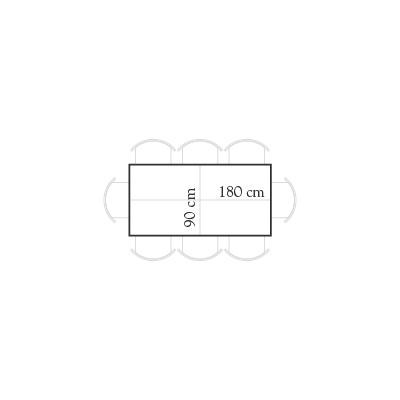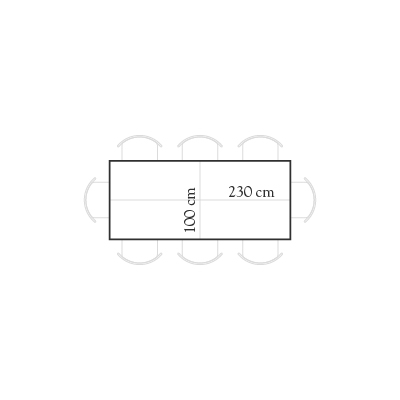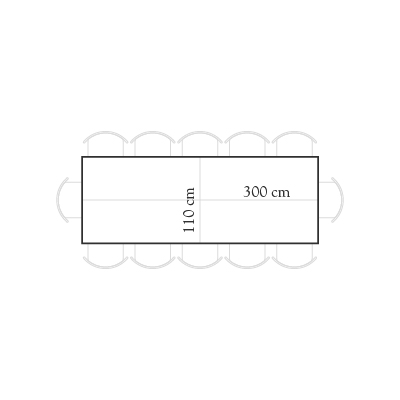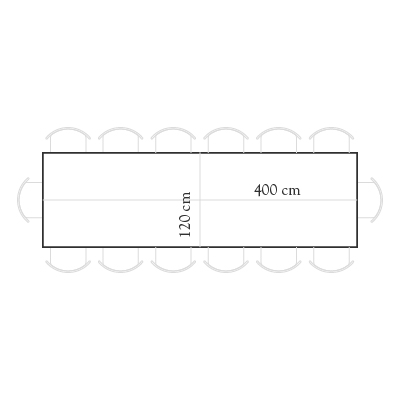## Square tables

A square table can have a minimum size of 70x70 cm- They are usually used in bars and restaurants and can have a maximum size that can exceed even 140x140 cm. For an optimal seat at the table it is suggested to opt for a minimum side size of 90 cm so that diners have more freedom of movement and there is adequate space for glasses and bottles.

For square tables, the seating calculation must be made considering one side with a minimum space per diner of 70 cm and the other side with a minimum space per diner of 60 cm. In this way the number of seats for a square table is optimal, giving sufficient space between adjacent diners in the corners.

Let's take for example a square table 130x130 cm.

• 130 / 70 = 1.85 which we round to 1
• 130 / 60 = 2.16 which we round to 2
• Number of seats = 1 + 1 + 2 + 2 = 6

Carrying out the same calculation for a 70x70 cm square table, the result is a number of seats equal to 4. For this measurement we prefer to indicate a number of seats equal to 2 for a comfort factor; the space for 2 diners allows you to have more availability and more freedom of movement above the table top.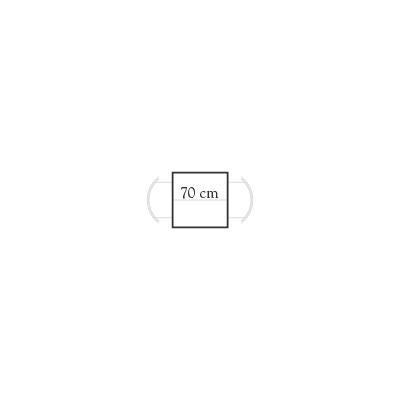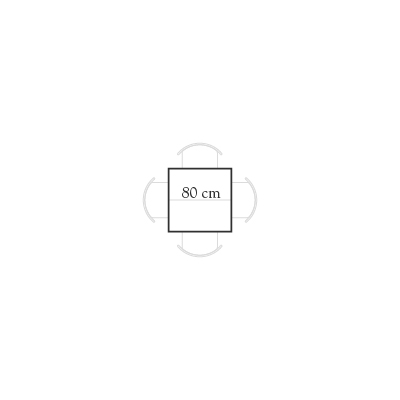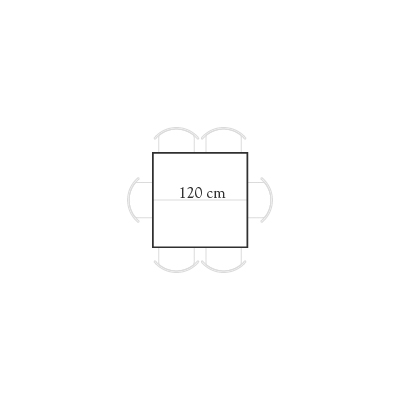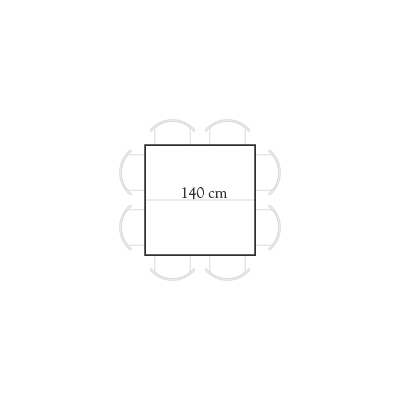## Round tables

The round table is an ideal solution to have all the table companions in front of each other, facilitating the conversation that can be shared by all participants. The round tables allow a better distribution of the tabe companions around the perimeter, guaranteeing the correct comfort with a suitable space for movement.

To calculate the number of seats in the round tables, it is necessary to consider the minimum space needed for each diner which is 60 cm along the circumference. The measurement of the circumference is obtained by multiplying the diameter of the table by the mathematical constant Pi (3.14).

Let's take for example a round table Ø 140 cm.

• 140 * 3.14 = 439.6 cm
• 439.6 / 60 = 7.33 which we round to 7
• Number of seats = 7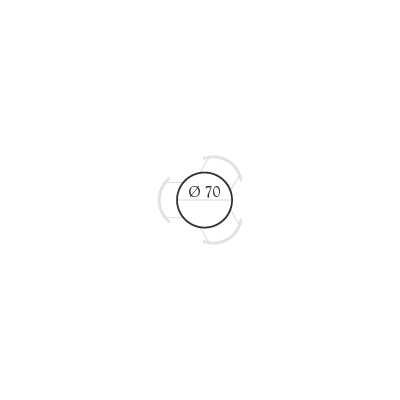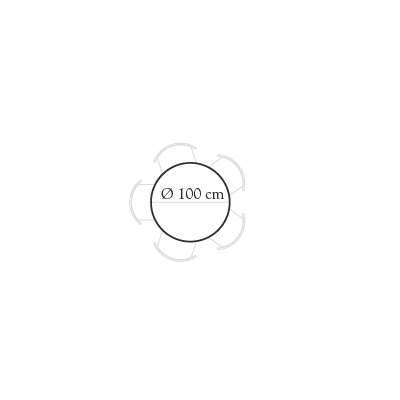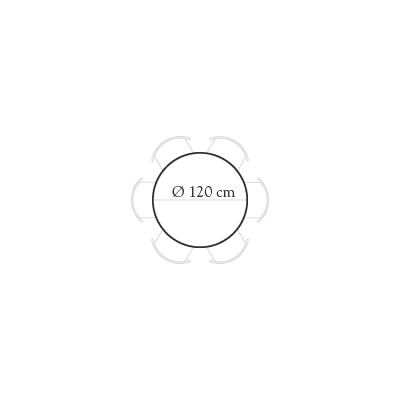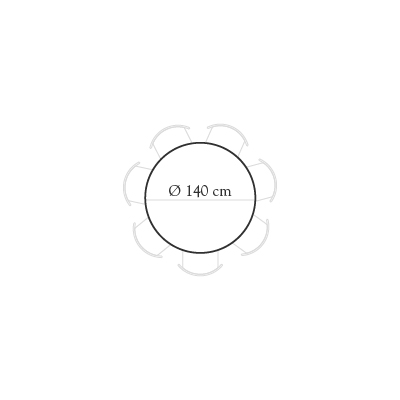## Elliptical tables / Oval tables

An elliptical table or an oval table is characterized by having a long side and a short side without any corner. This feature blends the advantages of a rectangular table and a round table, being able to accommodate many table companions by arranging them on one side to the other, favoring a collective dialogue.

The number of seats around an elliptical table is determined by the space needed by a diner which is 60 cm around the circumference. To calculate the perimeter of an oval table we need to perform a mathematical calculation: (2 * π) * [square root of [ ( (long axis / 2)² + (short axis / 2)² ) / 2].

In elliptical tables for aesthetic reasons it is advisable to always have an even number of chairs around the table. This reason is enhanced by the fact that if an oval table has a very narrow curvature at the head of the table, the diner would not have the right ergonomic space to sit comfortably.

Let's consider a 220x110 cm oval table.

• 2 * 3.14 = 6.28
• [(220/2)² + (110/2)²] / 2 = 7562.5
• Square root of 7562.5 = 86.96
• Table perimeter = 86.96 * 6.28 = 546.10 cm
• 546.10 / 60 = 9.1 which we round to 8
• Number of seats = 8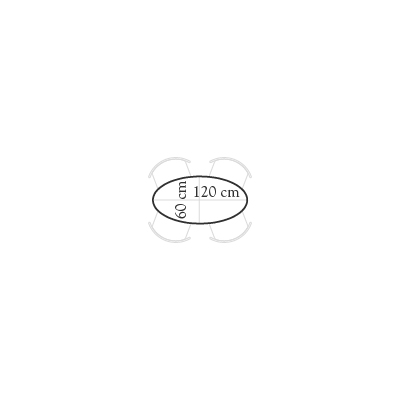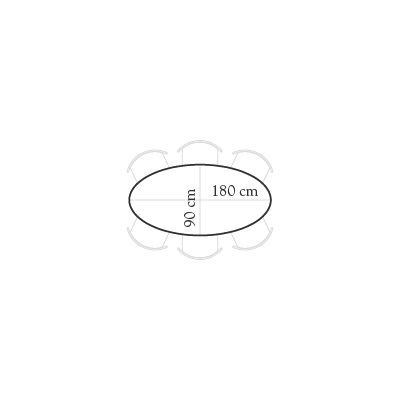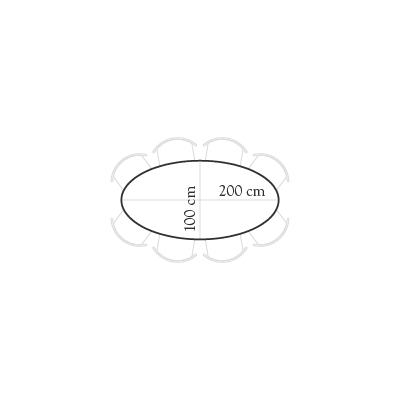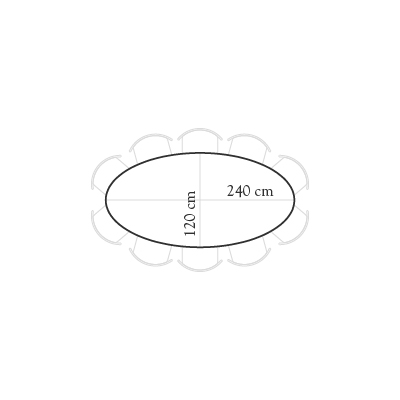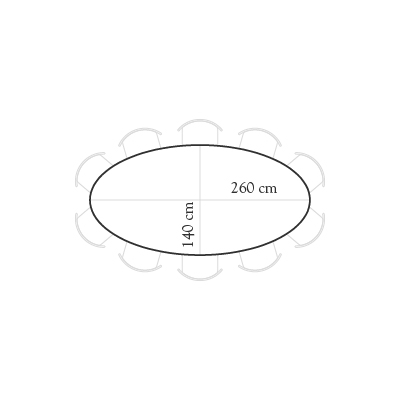Our customer service is available for any advice on the amount of chairs to accommodate a table. With the assistance of one of our interior designers, you will find the ideal solution for furnishing the dining room with table and chairs.